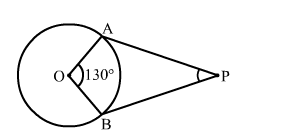# If the angle between two radii of a circle is 130°,`
Question:

If the angle between two radii of a circle is 130°, then the angle between the tangent at the ends of the radii is

(a) 65°
(b) 40°
(c) 50°
(d) 90°

Solution:(c) $50^{\circ}$

$\mathrm{OA}$ and $\mathrm{OB} a$ re the two $\mathrm{r}$ adii of $a$ circle with centre $\mathrm{O}$.

Also, $A P$ and $B P$ are the tangents to the circle.

Given, $\angle A O B=130^{\circ}$

Now, $\angle O A B=\angle O B A=90^{\circ}$ (S ince tangents drawn from an external point a re

perpendicular to the radius at point of contact)

In quadrilateral $O A P B$,

$\Rightarrow 130^{0}+90^{0}+90^{0}+\angle A P B=360^{0}$

$\Rightarrow \angle A P B=360^{0}-\left(130^{0}+90^{0}+90^{0}\right)$

$\Rightarrow \angle A P B=360^{0}-310^{\circ}$

$\Rightarrow \angle A P B=50^{\circ}$